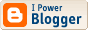## Note on the probability of century

This is mostly for myself so that I have something to refer to in future. In the previous post I skipped some algebra and gave a formula for the probability of a century, given a hazard function which is 3/(avg+3) on nought and 1/(avg+3) for all scores greater than nought.

The algebra I originally posted was slightly wrong (I have corrected it in the second edit), but could have been simplified. The probability of a score of at least 100 is [avg/(avg+3)] * [(avg+2)/(avg+3)]99. It is a little bit annoying having such a high exponent. Re-write (avg+2)/(avg+3) as [1-1/(avg+3)]. Then from a definition of the exponential function, raising that to the power of 99 is approximately equal to exp[-99/(avg+3)].

So, the probability of a century is [avg/(avg+3)] * exp[-99/(avg+3)].

Empirically, the simple exp(-100/avg) is a better predictor of the fraction of a batsman's innings that are centuries. If we exclude not-outs below 100, then it is much of a muchness between those two and exp(-99/avg).Subscribe to Posts [Atom]